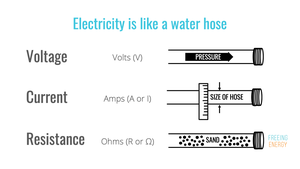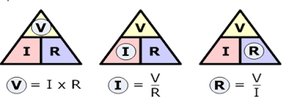# Limitations and Applications of Ohm’s Law

• Last Updated : 22 Sep, 2021

Ohm’s law is a relationship between three physical phenomena: current, voltage, and resistance. This relationship was introduced by German physicist George Simon Ohm. That is why the law is well known as Ohm’s law. It states that the amount of steady current through a large number of materials is directly proportional to the potential difference, or voltage, across the materials. Thus, if the voltage V (in units of volts) between two ends of a wire made from one of these materials is tripled, the current I (in amperes) also triples; and the quotient V/I remains constant. The quotient V/I for a given piece of material is called its resistance, R, measured in units named ohms.

• Current: Current is defined as the flow of positive charge from a source to a negative charge source. The units for current are C/s for the amount of charge (C/Coloumb) that travels per unit time (second). The ampere (A) is the common unit of current equal to 1 C/s and the symbol for current is I.
• Voltage: Voltage is the driving force of the current, it is the electromotive force that pushes free electrons from one atom to another in the same direction. It is also known as potential difference. Voltage is measured by the unit Volt(V).
• Resistance: The electrical resistance of an object is a measure of its opposition to the flow of electric current. Its reciprocal quantity is electrical conductance. The unit of resistance is Ohm.Attention reader! All those who say programming isn't for kids, just haven't met the right mentors yet. Join the  Demo Class for First Step to Coding Course, specifically designed for students of class 8 to 12.

The students will get to learn more about the world of programming in these free classes which will definitely help them in making a wise career choice in the future.

For any given the current, voltage, and resistance, Ohm’s law is defined as:

V = I·R

### Statement of Ohm’s Law

At constant temperature, the current through an ideal resistor is directly proportional to the voltage applied across the resistor. The constant of proportionality is written as R and this is the resistance value of the resistor.The main criteria for Ohm’s law are to keep the resistance constant because proportionality constant in the relationship is resistance R. But we know that the variation of temperature affects the value of resistance so to keep the resistance constant during experiments of Ohm’s law the temperature is considered constant.

### Limitations of Ohm’s Law

1. Ohm’s law is not applicable for unilateral electrical elements like diodes and transistors as they allow the current to flow through in one direction only.
2. For non-linear electrical elements with parameters like capacitance, resistance, etc. the voltage and the current won’t be constant with respect to time making it difficult to use Ohm’s law. Non-linear elements are those which do not have current exactly proportional to the applied voltage, which means the resistance value of those elements changes for different values of voltage and current. Examples of non – linear elements are thyristor, electric arc, etc.
3. The relation between V and I depends on the sign of V(+ or -). In other words, if I is the current for a certain V, then reversing the direction of V keeping its magnitude fixed, does not produce a current of the same magnitude as I in the opposite direction. This happens for example in the case of a diode.
4. Ohm’s law is only applicable in metallic conductors. So it won’t work in the case of non-metallic conductors.

### Applications of Ohm’s law in Daily Life

Ohm’s law can determine the voltage applied in a circuit, the value of resistance, and the current flowing through the circuit. With the help of the above three values, we can find the value of other factors like resistivity and many more. Some daily applications of Ohm’s law:

• In fuses: In order to protect a circuit, fuses and circuit breakers are used. These are connected in series with the electrical appliances. Ohm’s law allows us to find the value of the current which could flow through the fuses. If the current value is too large, then it could damage the circuit and even lead to the explosion of the electronic device.
• To know power consumption: The electrical heaters have a high-resistance metal coil that allows a certain amount of current to pass across them to provide the heat needed. Using this law, the power to be given to the heaters is determined.
• To control the speed of fans: By shifting the regulator to the end from start, we can regulate the speed of the fans in our houses. By controlling the resistance via the regulator, the current flowing through the fan is managed here. We can measure the resistance, current, and thus power flowing via Ohm’s Law for any particular value of the input.
• For deciding the size of resistors: Electric appliances like electric kettles and irons have a lot of resistors inside them. In order to provide the necessary amount of heat, the resistors restrict the amount of current that can flow through them. By using Ohm’s law, the size of resistors included in them is defined.

### Sample Problems

Problem 1: What is the current flowing in a 75 W light bulb connected to 120 V?

Solution:

We have given the value of power (P = 75W) and value of Voltage (V = 120V).

We want to find the value of current I.

Using Ohm’s law,

P = IV

or

I = P / V

= 75 / 120

= 0.625 A.

Problem 2: If the resistance of an electric iron is 100 Ω and a current of 4.2 A flows through the resistance. Find the voltage between two points.

Solution:

If we are asked to calculate the value of voltage with the value of current and resistance given to us, then cover V in the triangle. Now, we are left with I and R or more precisely I × R.

Therefore, we use the following formula to calculate the value of V:

V = I × R

Substituting the values in the equation, we get

V = 4.2 A × 100  = 420 V

V = 420 V

Problem 3: An EMF source of 10.0 V is connected to a purely resistive electrical appliance. An electric current of 1.0 A flows through it. Consider the conducting wires to be resistance-free. Calculate the resistance offered by the electrical appliance.

Solution:

When we are asked to find out the value of resistance when the values of voltage and current are given, then we cover R in the triangle. This leaves us with only V and I, more precisely V / I.

Substituting the values in the equation, we get

R = V / I

R = 10 V / 1 A = 10 Ω

R = 10 Ω

Problem 4: Find the current I through a resistor of resistance R = 3 Ω if the voltage across the resistor is 9 V.

Solution:

Substitute R by 2 and V by 6 in Ohm’s law V = R I.

9 = 3 I

Solve for I,

I = 9 / 3 = 3 A

Problem 5: In the circuit below resistors R1 and R2 are in series and have resistances of 5 Ω and 10 Ω, respectively. The voltage across resistor R1 is equal to 4 V. Find the current passing through resistor R2 and the voltage across the same resistor.

Solution:

We use Ohm’s law V = R I to find the current I1 passing through R1.

4 = 5 I1

Solve for I1

I1 = 4 / 5 = 0.8 A

The two resistors are in series and therefore the same current passes through them. Hence the current I2 through R2 is equal to 0.8 A.

We now use Ohm’s law to find the voltage V2 across resistor R2.

V2 = R2 I2 = 10 (0.8) = 8 V

Problem 6: In the circuit below resistors R1 and R2 are in parallel and have resistances of 8 Ω and 4 Ω, respectively. The current passing through R1 is 0.2 A. Find the voltage across resistor R2 and the current passing through the same resistor.

Solution:

Use Ohm’s law V = R I to find the voltage V1 across resistor R1.

V1 = 8 (0.2) = 1.6 V

The voltage across resistor R1 and the voltage across resistor R2 are the same because R1 and R2 are in parallel.

We now use Ohm’s law to find current I2 passing through resistor R2.

1.6 = 4 I2

Solve for I2

I2 = 1.6 / 4 = 0.4 A

Problem 7: The current passing through a resistor in a circuit is 0.01 A when the voltage across the same resistor is 5 V. What current passes through this resistor when the voltage across it is 7.5 V?

Solution:

Use Ohm’s law V = R I to find the resistor R in this circuit.

5 = R (0.01)

Solve for R

R = 5 / 0.01 = 500 Ω

We now use Ohm’s law V = R I and the value of R to find the current when the voltage is 7.5.

7.5 = 500 I

Solve for I

I = 7.5 / 500 = 0.015 A

Problem 8: Find the current of an electrical circuit that has a resistance of 100 Ohms and a voltage supply of 10 Volts.

Solution:

Given that,

V = 10 V

R = 100 Ω

Therefore,

I = V / R

= 10 V / 100 Ω

= 0.1 A

= 100 mA

My Personal Notes arrow_drop_up